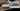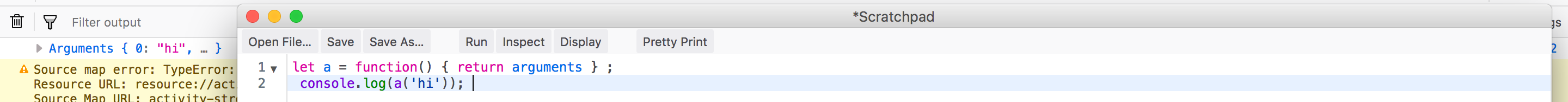back to all posts

# Interview Preparation - Tricky Questions - 2

#### by Nabendu Biswas / November 14th, 2018

##### Series: Interview-webdevWelcome to part-16 of the series and the second part in Tricky questions.

Question 109- What is the output of the below code and why?

``````    (function(x) {
return (function(y) {
console.log(x);
})(2)
})(1);
``````

Answer-The output logged will be 1 and that will be because of closure. Here we have an outer IIFE and an inner IIFE. First the outer IIFE will run and will set x=1. Now when the inner IIFE will run, it will remember the value of “x” because of closure and console log will dispaly 1.

Question 110- What is the output of the below code and why?

``````    for (let i = 0; i < 5; i++) {
setTimeout(function() { console.log(i); }, i * 1000 );
}
``````

``````    0
1
2
3
4
``````

It will output the above because we use let instead of var here. The variable i is only seen in the for loop’s block scope.

Question 111- What is the output of the below code and why?

``````    var a = [1, 2, 3];
a = 99;
console.log(a);
``````

Answer-The output logged will be: undefined

When we give the statement `a = 99;` the JavaScript engine will make array slots 3 through 9 be “empty slots.”

So, `a` will give undefined

Question 112- What is the output of the below code and why?

``````    console.log(typeof undefined == typeof NULL);
``````

Answer-The output logged will be: true

The reason is that NULL is different from primitive data type null. The variable NULL is just any other undefined variable. If we console log these three, we can see that typeof null and typeof NULL are different.

``````    console.log(typeof undefined); **//undefined**
console.log(typeof null);  **//object**
console.log(typeof NULL);  **//undefined**
``````

Question 113- What is the output of the below code and why?

``````    console.log(typeof typeof 1);
``````

Answer-The output logged will be: string

The reason is that typeof 1 will return "number" and typeof "number" will return string.

Question 114- What is the output of the below code and why?

``````    var b = 1;
function outer(){
var b = 2
function inner(){
b++;
var b = 3;
console.log(b)
}
inner();
}
outer();
``````

Answer-The output logged will be: 3

There are three closures in the example, each with it’s own var b declaration. When a variable is invoked closures will be checked in order from local to global until an instance is found. Since the inner closure has a b variable of its own, that is what will be output.

Question 115- What is the output of the below code and why?

``````    console.log([] + []);
``````

Answer-The output logged will be: `“ “` ie two empty spaces.

The reason is the plus(+) operator works on numbers and strings. So, when we give two empty arrays, it will typecast them to empty string and then print them.

Question 116- What is the output of the below code and why?

``````    function a(greet) {
return greet;
}

const sentence = a `hi`;

console.log(sentence);
``````

Answer-The output logged will be: `Array ["hi"]`

The reason is the the line const sentence = a `hi`; also passes an argument to the function and it is know as tagged template. It is quite similar to the line const sentence = a(`hi`);

Question 117- How can you prevent user from adding new properties or modifying a property in an Object?

Answer-We can prevent any user from adding a new property or modifying existing property in an Object by using the Object’s method freeze(). Below is the usage.

``````    let profile = {
name: "Nabendu"
};

Object.freeze(profile);

profile.age = 30;
profile.name = "Shikha";
console.log(profile); //**Object { name: "Nabendu" }**
``````

Question 118- How can you prevent user from adding new properties but can modify an existing property in an Object?

Answer-We can prevent any user from adding a new property but allow to modify existing property in an Object by using the Object’s method seal(). Below is the usage.

``````    let profile = {
name: "Nabendu"
};

Object.seal(profile);

profile.age = 30;
profile.name = "Shikha";

console.log(profile); //**Object { name: "Shikha" }**
``````

Question 119- What is the output of the below code and why?

``````    let x = [1, 2, 3] + [4, 5, 6];

console.log(x);
``````

Answer-The output will be `1,2,34,5,6`

It will be so, because the plus(+) operator works only on Numbers and Strings. So, in above question it will try to convert the arrays first. Since, they cannot be converted into Number, so they will be converted into a Strings like "1,2,3" + "4,5,6". And this string concatenation will produce "1,2,34,5,6"

Question 120- What is the output of the below code and why?

``````    console.log(888888888888888888);
``````

The reason is that in JavaScript we cannot have a number more then 16 digit. The question have 8 for 18 times. The largest number available in JavaScript is Number.MAX_SAFE_INTEGER. If we console log it, we will find that it have 16 digit.

``````    console.log(Number.MAX_SAFE_INTEGER); **//9007199254740991**
``````

Question 121- What is the differene between async and defer attributes in script tag?

Answer-In JavaScript when the parser reaches the `<script>` tag, it stops to fetch it(if external file) and run it.

This can be a problem, because it generally stops our html code been rendered to the DOM and visible to the user. One way to solve this issue is to put the `<script>` tag just before the body tage ends ie `</body>`. But in some cases we might require some features from our javascript to be available to the page.

We will look at the three type of execution here — Normal, async and defer.

Normal Execution Take the example of `<script>` been located somewhere middle of the body.

``````    <html>
<body>
...
<script src="script.js">
....
</body>
</html>
``````

As mentioned earlier, the HTML parsing will be stop to fetch and then execute the script.Normal Execution

Async Execution The async attribute in the script tag indicates to the browser that script file can be executed asynchronously.

``````    <html>
<body>
...
<script async src="script.js">
....
</body>
</html>
``````

The script is fetched on parallel with the HTML parsing. But once the script is fetched, the HTML parsing is paused to execute the script.Async Execution

Defer Execution The defer attribute in the script tag indicates to the browser that script file to be executed only once the HTML parsing is completed.

``````    <html>
<body>
...
<script defer src="script.js">
....
</body>
</html>
``````

Like async the script can be fetched in parallel to HTML parsing. But even if the script is fetched before the HTML parsing is completed, it will wait for the parsing to be completed before executing the script.Defer Execution

Question 122- What is the output of the below code and why?

``````        console.log(false == ‘0’)
console.log(false === ‘0’)
``````

``````        true
false
``````

In JavaScript the == does type conversion before comparing, but === doesn’t do type conversion. The explanation is as below:

Eg 1: As we know JavaScript have it’s set of truthy and falsy values. Details of it can be found here. So, ‘0’ is a truthy value. But when you explicitly do false == ‘0’, both sides are being converted to numbers, and then the comparison is performed. So, it will become 0 == 0 and result in true.

Eg 2: As stated earlier the === doesn’t do type conversion and so will result in false.

Question 123- What is the output of the below code and why?

``````    var x = 21;
var girl = function () {
console.log(x);
var x = 20;
};
girl ();
``````

``````    undefined
``````

It is because in JavaScript there is a compiler and interpreter step. So, when the compiler runs, it will register “x” in girl’s scope. But when the interpreter comes to console.log(x) , it gets from above interpreter step that “x” is in girl’s scope. But as it doesn’t have any value to it so undefined is printed.

You can go through my earlier blog here to understand how the compiler and interpreter step runs.

Question 124- What is the output of the below code and why?

``````    console.log(1 < 2 < 3);

console.log(3 > 2 > 1);
``````

``````    true
false
``````

The explanation is below: Eg 1: The JavaScript engine compares left to right. So 1 < 2, will give true. Then the statement will become true < 3. Now to compare it true will be changed into 1 and then 1 < 3will give true Eg 2: The comparison 3 > 2 will give true. Then the statement will become true > 1. Now to compare it true will be changed into 1 and then 1 > 1will give false

Question 125- What is the output of the below code and why?

``````        let a = () => arguments;
console.log(a(‘hi’));
``````

Answer-To understand it, let’s change the arrow function to a regular function. The normal function have access to arguments object, which is an array like object and returns the number of arguments passed.arguments in normal function

Now, back to the arrow function. The arrow function doesn’t have access to argument object. So, if we run the arrow function code, it will result in Reference error.

``````    /*
Exception: ReferenceError: arguments is not defined
*/
``````

Question 126- What is the output of the below code and why?

``````    const x = [1, 2, 3];
x[-1] = -1;
console.log(x[x.indexOf(999)]);
``````

Answer-The output logged will be: -1

The reason is that we have already declared `x[-1]` as -1. The array function indexOf is used to find element in an array. If the element is not found it returns -1. Now x.indexOf(999), will give -1 because there is no such item as 999 in the array. So, we are effectively console logging `x[-1]` which is -1.

This concludes the part-16 of the series and final part of Tricky questions.